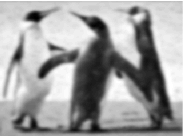# MATLAB – Butterworth Lowpass Filter in Image Processing

In the field of Image Processing, Butterworth Lowpass Filter (BLPF) is used for image smoothing in the frequency domain. It removes high-frequency noise from a digital image and preserves low-frequency components. The transfer function of BLPF of orderis defined as-Where,

•is a positive constant. BLPF passes all the frequencies less thanvalue without attenuation and cuts off all the frequencies greater than it.
• Thisis the transition point between H(u, v) = 1 and H(u, v) = 0, so this is termed as cutoff frequency. But instead of making a sharp cut-off (like, Ideal Lowpass Filter (ILPF)), it introduces a smooth transition from 1 to 0 to reduce ringing artifacts.
•is the Euclidean Distance from any point (u, v) to the origin of the frequency plane, i.e,Approach:
Step 1: Input – Read an image
Step 2: Saving the size of the input image in pixels
Step 3: Get the Fourier Transform of the input_image
Step 4: Assign the orderand cut-off frequencyStep 5: Designing filter: Butterworth Low Pass Filter
Step 6: Convolution between the Fourier Transformed input image and the filtering mask
Step 7: Take Inverse Fourier Transform of the convoluted image
Step 8: Display the resultant image as output

Implementation in MATLAB:

 % MATLAB Code | Butterworth Low Pass Filter         % Reading input image : input_image  input_image = imread('[name of input image file].[file format]');     % Saving the size of the input_image in pixels-  % M : no of rows (height of the image)  % N : no of columns (width of the image)  [M, N] = size(input_image);     % Getting Fourier Transform of the input_image  % using MATLAB library function fft2 (2D fast fourier transform)  FT_img = fft2(double(input_image));     % Assign the order value  n = 2; % one can change this value accordingly     % Assign Cut-off Frequency  D0 = 20; % one can change this value accordingly     % Designing filter  u = 0:(M-1);  v = 0:(N-1);  idx = find(u > M/2);  u(idx) = u(idx) - M;  idy = find(v > N/2);  v(idy) = v(idy) - N;     % MATLAB library function meshgrid(v, u) returns   % 2D grid which contains the coordinates of vectors   % v and u. Matrix V with each row is a copy of v   % and matrix U with each column is a copy of u   [V, U] = meshgrid(v, u);     % Calculating Euclidean Distance  D = sqrt(U.^2 + V.^2);     % determining the filtering mask  H = 1./(1 + (D./D0).^(2*n));     % Convolution between the Fourier Transformed   % image and the mask  G = H.*FT_img;     % Getting the resultant image by Inverse Fourier Transform   % of the convoluted image using MATLAB library function    % ifft2 (2D inverse fast fourier transform)     output_image = real(ifft2(double(G)));        % Displaying Input Image and Output Image   subplot(2, 1, 1), imshow(input_image),   subplot(2, 1, 2), imshow(output_image, [ ]);

Input Image –Output:Note: A Butterworth filter of order 1 has no ringing artifact. Generally ringing is imperceptible in filters of order 2. But it can become a significant factor in filters of a higher order. For a specific cut-off frequency, ringing increases with an increase in the filter order.

My Personal Notes arrow_drop_upCheck out this Author's contributed articles.

If you like GeeksforGeeks and would like to contribute, you can also write an article using contribute.geeksforgeeks.org or mail your article to contribute@geeksforgeeks.org. See your article appearing on the GeeksforGeeks main page and help other Geeks.

Please Improve this article if you find anything incorrect by clicking on the "Improve Article" button below.

Article Tags :

Be the First to upvote.

Please write to us at contribute@geeksforgeeks.org to report any issue with the above content.